# SAT II Math II : Other Properties

## Example Questions

### Example Question #51 : Functions And Graphs

Define the functionsand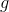on the set of real numbers as follows: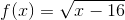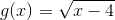Give the natural domain of the composite function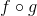.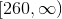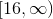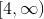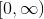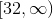Explanation:

The natural domain of the composite functionis defined to be the intersection of the two sets.

One set is the natural domain of. Sinceis defined to be the square root of an expression, the radicand must be nonnegative. Therefore,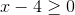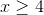This set is.

The other set is the set of numbers that the functionpairs with a number within the domain of. Since the radicand of the square root inmust be nonnegative,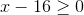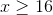For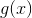to fall within this set: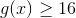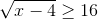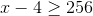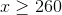This set is.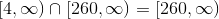, which is the natural domain.